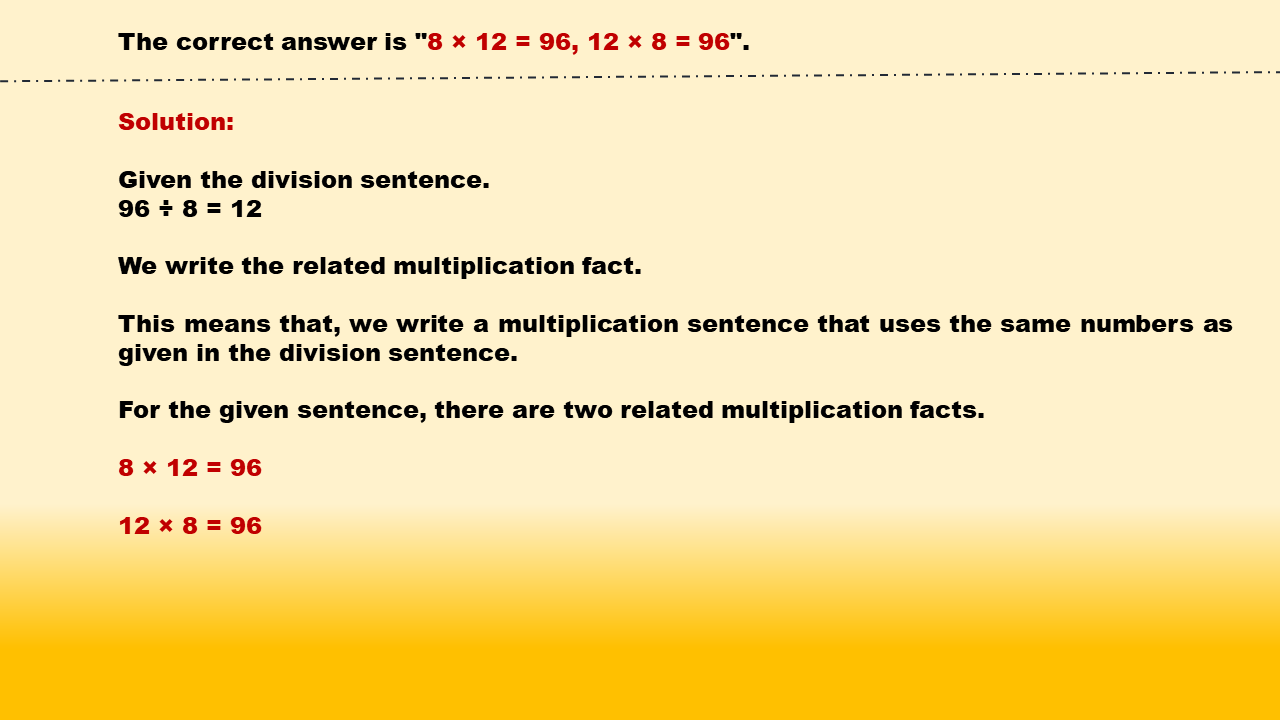1
visibility

Identify related multiplication facts for 96 ÷ 8 = 12.

• 8 × 7 = 56, 7 × 8 = 56

• 8 × 5 = 40, 5 × 8 = 40

• 8 × 12 = 96, 12 × 8 = 96

• 8 × 14 = 112, 14 × 8 = 112